# How to fix Plotly Python putting a bar chart in front of trace lines.

in Programming & Dev3 years ago

I'm posting this in case it helps anyone else. It caused me 4 hours of horrible frustration.

I'm trying draw a quite hideously over complicated set of graphs which I've cobbled together in truly apocalyptically bad spaghetti code in Python. But it kinda works.

And then I added a bar graph that I needed to go behind the lines. This is what happened.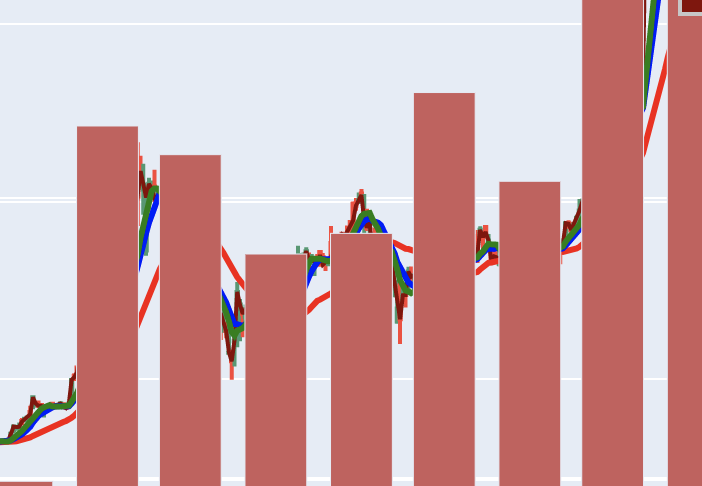One day I'll share more of the full graphs, suffice it to say they're being prepared for our next #CryptoClassAction court date in March.

This particular graph has two subplots one above the other with 4 y-axis, 2 on each subplot.

I reduced my problem to a much simpler example: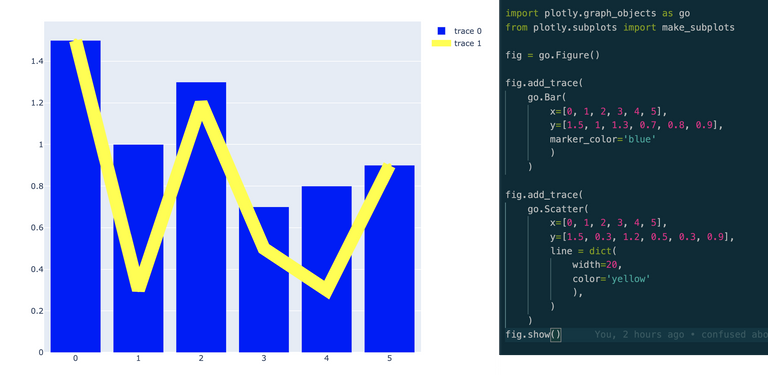The code for which looks like this:

``````import plotly.graph_objects as go
from plotly.subplots import make_subplots

fig = go.Figure()

go.Bar(
x=[0, 1, 2, 3, 4, 5],
y=[1.5, 1, 1.3, 0.7, 0.8, 0.9],
marker_color='blue'
)
)

go.Scatter(
x=[0, 1, 2, 3, 4, 5],
y=[1.5, 0.3, 1.2, 0.5, 0.3, 0.9],
line = dict(
width=20,
color='yellow'
),
)
)
fig.show()
``````

So far so good. Then I converted this to a plot with subplots and separate y-axis and mimicked what I did with my complex plot: I ADDED the bar graph and created a secondary y-axis for it. Here's the graph I got and the code: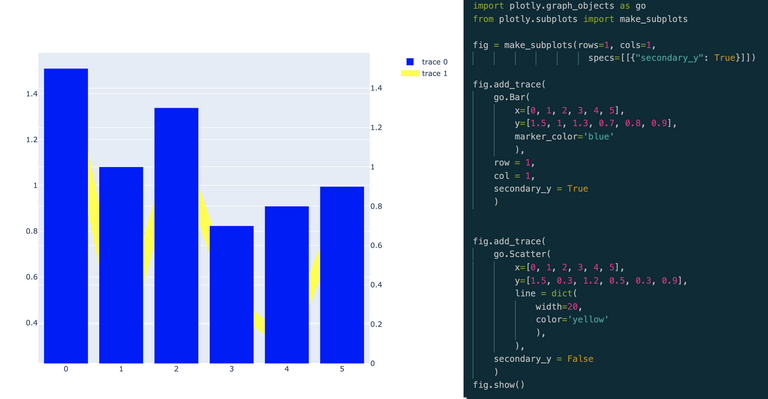``````import plotly.graph_objects as go
from plotly.subplots import make_subplots

# fig = go.Figure()
fig = make_subplots(rows=1, cols=1,
specs=[[{"secondary_y": True}]])

go.Bar(
x=[0, 1, 2, 3, 4, 5],
y=[1.5, 1, 1.3, 0.7, 0.8, 0.9],
marker_color='blue'
),
row = 1,
col = 1,
secondary_y = True
)

go.Scatter(
x=[0, 1, 2, 3, 4, 5],
y=[1.5, 0.3, 1.2, 0.5, 0.3, 0.9],
line = dict(
width=20,
color='yellow'
),
),
secondary_y = False
)
fig.show()
``````

And immediately I got the idea... the only thing I needed to do was switch over the secondary_y axis. For some reason I can't figure out in the Plotly system, secondary_y axes items always overwrite the primary. Here's the final graph and the code.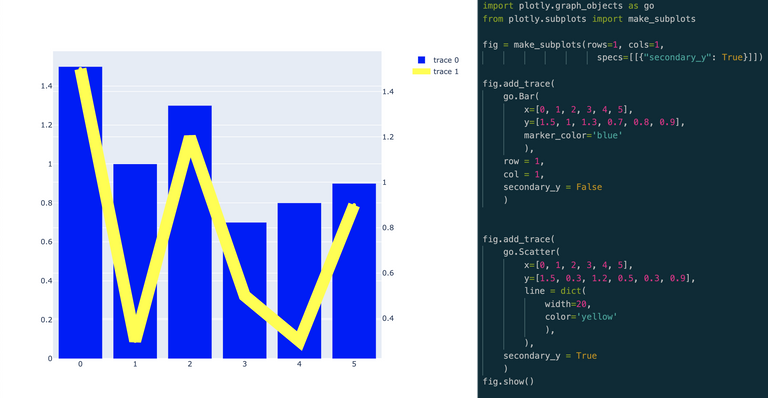All I had to do was flip the bar graph onto the first y-axis and the lines onto the secondary y-axis. I've done that now on my main graph and voila... it worked.

This isn't a finished product yet, it needs a fair bit of work, but here's the current draft.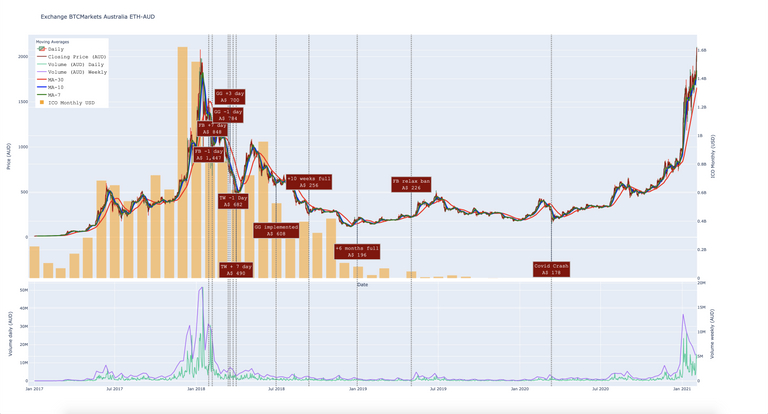You should be able to play with the interactive HTML version at this link.

Thanks for looking and I hope this helps someone one day!

Sort:

I've always wanted to get started in making charts, and thing might just give me a reason to start.

Amazing work preparing all these charts for the #cryptoclassaction.

It is much appreciated.

Congratulations @brianoflondon! You have completed the following achievement on the Hive blockchain and have been rewarded with new badge(s) :You distributed more than 40000 upvotes. Your next target is to reach 41000 upvotes.

If you no longer want to receive notifications, reply to this comment with the word `STOP`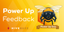Feedback from the February 1st Hive Power Up Day